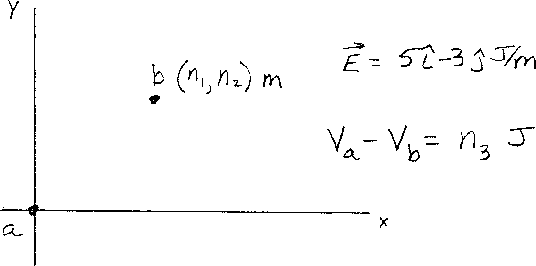Problem A10: In the figure above, there is a constant electric field given by E = ( 5 i - 3 j ) J/C/M. The point a is at the origin (0,0), and the point b is at the coordinates (n1,n2) meters in the x-y plane. What is n3 in the expression for the potential difference between point a and point b :V(a)-V(b)=n3 J/C.n1 = n2 = Input n3:

If you are currently in my class, you can record your grade by entering your name and student ID number (without the leading zeros) below and clicking on "record grade".
 First Name = Last Name = ID = Problem: